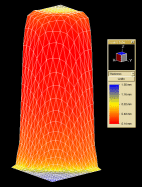B-SIM V2.5 Reference - Blow molding simulationPatran output

Output to Patran is the most advanced one. It creates five files enabling structural analysis and calculation of shrinkage and cooling. The exported files contain the geometry and the topological data along with nodal displacements, thicknesses, temperatures, residual stresses, extensions and direction cosines.

Files created using Patran output

 File extension Description *.ptn Contains original grid (sheet, preform/parison) nodal coordinates and topology *.nod Contains deformed grid (sheet, preform/parison) nodal displacements + thicknesses *.tep Contains deformed grid (sheet, preform/parison) nodal displacements + temperatures *.elm Contains deformed grid elements. Every element has listed: 1) Its number 2) Residual stresses Sigma1, Sigma2, Sigma3 (in principal direction) 3) Direction cosines (for local x and y axis) 4) Extensional ratios in principal directions (lambda1, lambda2) Here is a detailed description of the file format - on each row are the following data: Elem# Sigma1 Sigma2 Sigma3 vx_x vx_y vx_z vy_x vy_y vy_z lambda1 lambda2, where Elem# is element number Sigma1, Sigma2 and Sigma3 are principal stresses vx_x, vx_y, vx_z are components of the direction cosines of local x axis vy_x, vy_y, vy_z are components of the direction cosines of local y axis lambda1, lambda2 are principal extensions *.fin Contains final deformed grid (sheet, preform/parison) topology - this file has the same format as *.ptn except the nodal coordinates - they correspond to the deformed grid

Example

Exported grid: